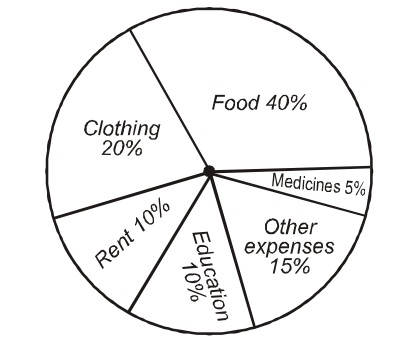## Pie chart

#### Data Interpretation

Direction: The pie-chart given below shows the household expenditure of a family on different items. Study the chart carefully and answer the questions that follows.1. If the family's expenditure on education is Rs. 375 then the same amount is being expent by this family on which of the following items?

1. Both segments representing education and rent respectively are each 10%.

##### Correct Option: C

Both segments representing education and rent respectively are each 10%. Hence, family's expenditure on these two items will be equal. i.e.. Rs. 375 will be expenditure on rent.

1. If the family expends Rs. 750 per month on food, what is the annual expenditure of this family on education?

1. Here as per graph food expenditure, 40% = Rs. 750
⇒ 10% = 10 x 750/40 = Rs. 187.50

##### Correct Option: C

Here as per graph food expenditure , 40% = Rs. 750 per month
⇒ 10% = 10 x 750/40 = Rs. 187.50 per month
As per graph 10% is the expenditure on education.
∴ Annual expenditure (12 months) of the family on education = 187.50 x 12 = Rs. 2250

1. If total expenditure of this family is Rs. 4500, find the expenditure of the family on clothing?

1. As per graph,
Expenditure on clothing = 20 % of total expenditure.

##### Correct Option: B

As per graph,
Expenditure on clothing = 20 %
Expenditure on clothing = Total expenditure x 20 %
Expenditure on clothing = 4500 x 20/100 = Rs. 900
Expenditure on clothing = 45 x 20 = Rs. 900

1. What is the angle subtended on the center by the segment representing other expenses?

1. As per graph,
other expenses = 15 % of total expenditure.

##### Correct Option: B

As per graph,
other expenses = 15 % of total expenditure.
As we know that the angle made on center in a circle is 360°
Since 100% made an angle 360° in a circle.
therefore 1% made an angle 360° / 100 in a circle.
therefore 15% made an angle 15 x 360° / 100 in a circle.
Central angle for 15% =15 x 36° / 10
Central angle for 15% =3 x 36° / 2
Central angle for 15% =3 x 18° = 54°

1. What is the ratio of total expenses on rent and clothing to the total expenses on medicines and other expenses of this family?

1. As per graph
Expenditure on rent and clothing = 10% + 20% = 30%
Expenditure on medicine and other expenses = 5% + 15% = 20%

##### Correct Option: D

As per graph
Expenditure on rent and clothing = 10% + 20% = 30%
Expenditure on medicine and other expenses = 5% + 15% = 20%
∴ Ratio = 30% : 20% = 3 : 2